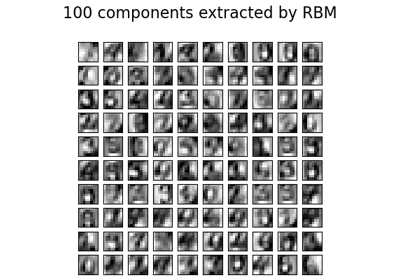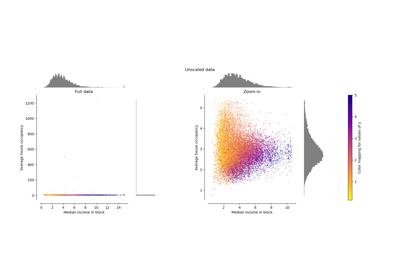# sklearn.preprocessing.minmax_scale¶

sklearn.preprocessing.minmax_scale(X, feature_range=(0, 1), *, axis=0, copy=True)[source]

Transform features by scaling each feature to a given range.

This estimator scales and translates each feature individually such that it is in the given range on the training set, i.e. between zero and one.

The transformation is given by (when axis=0):

X_std = (X - X.min(axis=0)) / (X.max(axis=0) - X.min(axis=0))
X_scaled = X_std * (max - min) + min


where min, max = feature_range.

The transformation is calculated as (when axis=0):

X_scaled = scale * X + min - X.min(axis=0) * scale
where scale = (max - min) / (X.max(axis=0) - X.min(axis=0))


This transformation is often used as an alternative to zero mean, unit variance scaling.

Read more in the User Guide.

New in version 0.17: minmax_scale function interface to MinMaxScaler.

Parameters:
Xarray-like of shape (n_samples, n_features)

The data.

feature_rangetuple (min, max), default=(0, 1)

Desired range of transformed data.

axis{0, 1}, default=0

Axis used to scale along. If 0, independently scale each feature, otherwise (if 1) scale each sample.

copybool, default=True

Set to False to perform inplace scaling and avoid a copy (if the input is already a numpy array).

Returns:
X_trndarray of shape (n_samples, n_features)

The transformed data.

Warning

Risk of data leak Do not use minmax_scale unless you know what you are doing. A common mistake is to apply it to the entire data before splitting into training and test sets. This will bias the model evaluation because information would have leaked from the test set to the training set. In general, we recommend using MinMaxScaler within a Pipeline in order to prevent most risks of data leaking: pipe = make_pipeline(MinMaxScaler(), LogisticRegression()).

MinMaxScaler

Performs scaling to a given range using the Transformer API (e.g. as part of a preprocessing Pipeline).

Notes

For a comparison of the different scalers, transformers, and normalizers, see: Compare the effect of different scalers on data with outliers.

## Examples using sklearn.preprocessing.minmax_scale¶Restricted Boltzmann Machine features for digit classification

Restricted Boltzmann Machine features for digit classificationCompare the effect of different scalers on data with outliers

Compare the effect of different scalers on data with outliers